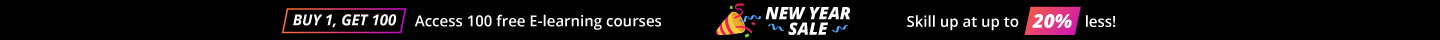# Data Science Interview Questions Data Science

This guide contains all the expert-designed data science interview questions you may expect when interviewing for a Data Scientist role. Following is the list of the Data Science interview questions and answers that is broken into 2 categories- Basic and Advanced interview questions and answers on Data Science.

• 4.6 Rating
• 45 Question(s)

## Beginner

An A/B test is a randomized experiment, where "A" and "B" refer to 2 variants, undertaken in order to determine which variant is the more "effective." A/B testing is a very celebrated method for figuring out the best online promotional and marketing strategies for your business. It can be used to test everything from website copy to sales emails to search ads. And the advantages A/B testing provide are enough to offset the additional time it takes.

One big caveat for A/B testing is “ beware of the results based on the small sample size”. Sample sizes for A/B testing is a tricky business, and not as straightforward as most think (or would hope). But this is really only one piece of a larger puzzle related to statistical confidence, which can only come with both the necessary number of samples and required time for the experiment to play out. Properly experiment design will take into account the number of samples and conversions required for a desired statistical confidence, and will allow the experiment to play out fully, without pulling the plug ahead of time because there appears to be a winner.

A categorical variable (sometimes called a nominal variable) is one that has two or more categories, but there is no intrinsic ordering to the categories.  For example, gender is a categorical variable having let’s say two categories (male and female) and there is no intrinsic ordering to the categories.  Hair colour is also a categorical variable having a number of categories (blonde, brown, brunette, red, etc.) and again, there is no agreed way to order these from highest to lowest.  A purely categorical variable is one that simply allows you to assign categories but you cannot clearly order the variables.  If the variable has a clear ordering, then that variable would be an ordinal variable, as described below.

1. Ordinal Variable - An ordinal variable is similar to a categorical variable.  The difference between the two is that there is a clear ordering of the variables.
2. Interval Variable - An ordinal variable is similar to a categorical variable.  The difference between the two is that there is a clear ordering of the variables.

Why does it matter if a variable is categorical, ordinal or interval?

Statistical computations and analyses assume that the variables have specific levels of measurement.  For example, it would not make sense to compute an average hair colour.  An average of a categorical variable does not make much sense because there is no intrinsic ordering of the levels of the categories. Moreover, if you tried to compute the average of educational experience as defined in the ordinal section above, you would also obtain a nonsensical result. Because the spacing between the four levels of educational experience is very uneven, the meaning of this average would be very questionable.  In short, an average requires a variable to be interval. Sometimes you have variables that are “in between” ordinal and interval, for example, a five-point Likert scale with values “strongly agree”, “agree”, “neutral”, “disagree” and “strongly disagree”.  If we cannot be sure that the intervals between each of these five values are the same, then we would not be able to say that this is an interval variable, but we would say that it is an ordinal variable.  However, in order to be able to use statistics that assume the variable is interval, we will assume that the intervals are equally spaced.

Machine learning arises from this question: could a computer go beyond “what we know how to order it to perform” and learn on its own how to perform a specified task? Could a computer do things or learn as human being does? Rather than programmers crafting data-processing rules by hand, could a computer automatically learn these rules by looking at data?

“A machine-learning system is trained rather than explicitly programmed. It’s presented with many examples relevant to the task, and it finds statistical structure in these examples that eventually allows the system to come up with rules for automating the task. For instance, if you wished to automate the task of tagging your vacation pictures, you could present a machine-learning system with many examples of pictures already tagged by humans, and the system would learn statistical rules for associating specific pictures to specific tags.”

(Please refer to the Book – “Deep Learning with Python” by Francois Chollet)

Gradient descent is one of the most popular algorithms to perform optimization and widely used to optimize neural networks. At the same time, every state-of-the-art Deep Learning library contains implementations of various algorithms to optimize gradient descent (e.g. lasagne's, caffe's, and keras' documentation). Gradient descent is a way to minimize an objective function J(θ) parameterized by a model's parameters θ∈Rd by updating the parameters in the opposite direction of the gradient of the objective function ∇θJ(θ) w.r.t. to the parameters. The learning rate η determines the size of the steps we take to reach a (local) minimum. In other words, we follow the direction of the slope of the surface created by the objective function downhill until we reach a valley.

Batch gradient descent, computes the gradient of the cost function w.r.t. to the parameters θ for the entire training dataset:

θ=θ−η⋅∇θJ(θ)

As we need to calculate the gradients for the whole dataset to perform just one update, batch gradient descent can be very slow and is intractable for datasets that don't fit in memory. Batch gradient descent also doesn't allow us to update our model online, i.e. with new examples on-the-fly.

Stochastic gradient descent (SGD) in contrast performs a parameter update for each training example x(i) and label y(i):

θ = θ−η⋅∇θJ(θ; x(i); y(i))

Batch gradient descent performs redundant computations for large datasets, as it recomputes gradients for similar examples before each parameter update. SGD does away with this redundancy by performing one update at a time. It is therefore usually much faster and can also be used to learn online.

• Mini - Batch Gradient Descent -

Mini-batch gradient descent considers the best of both worlds and performs an update for every mini-batch of n training examples:

θ=θ−η⋅∇θJ(θ ; x(i:i+n) ; y(i:i+n))

This way, it a) helps in reducing the variance of the parameter updates, which can lead to more stable convergence; and b) can make an effective use of highly-optimized matrix optimizations common to state-of-the-art deep learning libraries that make computing the gradient w.r.t. a mini-batch very efficient. Common mini-batch sizes range between 50 and 256, but can vary for different applications. Mini-batch gradient descent is typically the algorithm of choice when training a neural network and the term SGD usually is employed also when mini-batches are used.

P-value in the parlance of statistics can be defined as “Lowest level of probability at which the null hypothesis can be rejected”. For key statistics like t-stat, P<=0.05 indicates that the underlying null hypothesis can be rejected in favour of alternative hypothesis at 5% level of significance and for p>0.05 indicates that we have less than absolute evidence that the null hypothesis is not true.

Linear Regression is the oldest, simple and widely used supervised machine learning algorithm for predictive analysis. It’s a method to predict a target variable by fitting the best linear relationship between the dependent and independent variable.The best fitting line can be found by making sure that the sum of all the distances between the shape and the actual observations at each point is as small as possible. The fit of the shape is “best” in the sense that no other position would produce less error given the choice of shape.

Types of Linear Regression:

1. Simple Linear Regression - This method uses a single independent variable to predict a dependent variable by fitting a best linear relationship.
2. Multiple Linear Regression - This method uses more than one independent variable to predict a dependent variable by fitting a best linear relationship.

## Intermediate

Response:

CRISP- DM stands for "Cross Industry Standard Process for Data Mining". This is a standard methodology used for end-to-end Data Science project or program execution. It follows various stages which involve different type of activities or tasks that are carried out during the program execution.

1. Business understanding – typical tasks include the following: determining business objective or goals of what needs to be accomplished, assessing the situation, determining data mining goals and trying to convert business problem into data problem, defining project plan with various tasks etc.
2. Data understanding – typical tasks include the following: collecting initial data, describing data, exploring data, verifying data quality etc. This helps in preparing exploratory data analysis and acts as an interim step to show what patterns, variations exist in the data and can be shown to respective stakeholders.
3. Data preparation – typical tasks include selecting specific data needed for modelling purposes, cleaning data, constructing data, integrating data and formatting data as needed per requirement and scope. Feature engineering is performed as part of this process step and prepared as an input to the next phase.
4. Modelling or Model development – typical tasks include selecting modelling techniques, generating test design, building model, assessing model etc. This phase is used to build models using various algorithms or methods.
5. Model evaluation – typical tasks include evaluating results, reviewing process, determining next steps etc. Various metrics are being used to evaluate multiple models or multiple experiments that were created as part of the previous step or phase.
6. Deployment – typical tasks include plan deployment, plan monitoring & maintenance, presenting product final report & reviewing the project etc. This refers to the operationalization phase of an existing model or solution which was created and evaluated as the best experiment to be elevated to the production environment for usage and consumption purposes.

These are iterative. Below diagram depicts a view of the process methodology.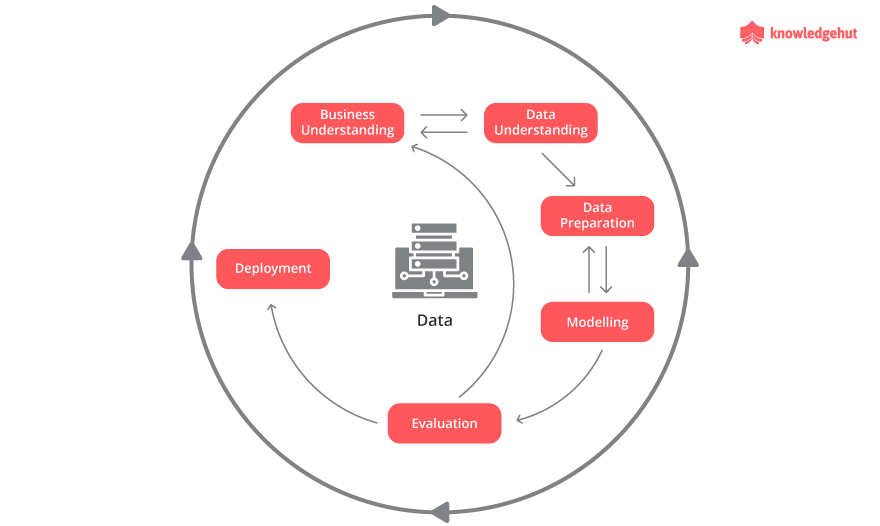Image Ref

Response:

The process of adding a tuning parameter to a model or algorithm to induce smoothness to prevent and address overfitting issues is called "Regularization". Regularization term is added to a mathematical equation to prevent the coefficients to fit perfectly,avoiding the risk of overfitting.

This is primarily performed by including a constant multiple to an existing weight vector. This constant is often either the L1 (Lasso) or L2 (Ridge), however, it can in actuality get into any norm. The model predictions should then minimize the mean of the loss or error function calculated on the regularized training set.

L1 or Lasso regularization helps perform feature selection in sparse feature spaces, and that is a good practical reason to use L1 in some situations. However, beyond that particular reason, L1 may not perform better than L2 in practice. Even in a situation where you might benefit from L1's sparsity to do feature selection, using L2 on the remaining variables is likely to give better results than L1 by itself.

Response:

There are multiple ways to make a model more robust to outliers, from different aspects either from data preparation perspective or from a model-building perspective.

An outlier is assumed as being unwanted, unexpected, or a must-be-incorrect value to the human's knowledge so far (e.g. no one can live longer than 150 years of age) rather than a rare event which is possible but rare. Outliers are usually defined as the sample distribution. Hence, outliers could be removed in the pre-processing step (before any learning phase happens), by using standard deviations(sd) such as (Mean +/- 2*sd), it can be used for normality. Otherwise, interquartile ranges from Q1 - Q3, where Q1 - is the "middle" value in the first half of the rank-ordered data set, Q3 - is the "middle" value in the second half of the rank-ordered data set. It can be used for not normal/unknown as threshold levels.

Below diagram shows typical outliers encircled with red circles for sample illustration purposes.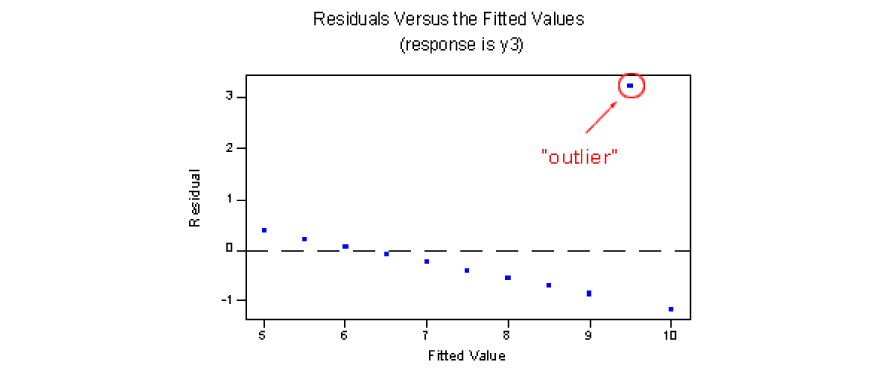Image Ref

Additionally, data transformation (e.g. log transformation) may help if data have a noticeable tail. When outliers are related to the sensitivity of the collecting instrument which may not precisely record small values, Winsorization may be useful. Winsorizing or winsorization is the transformation of statistics by limiting extreme values in the statistical data to reduce the effect of possibly spurious outliers.

This type of transformation has the same effect as clipping signals (i.e. replaces extreme data values with less extreme values). Another option to reduce the influence of outliers is using mean absolute difference rather mean squared error.

For model building purposes, some models are resistant to outliers (e.g. tree-based approaches) or non-parametric tests. Tree models typically divide each node into two parts in each split, which is similar to the median effect. Therefore, at each split, all data points in a bucket could be equally treated regardless of the extreme values they may have.

Response

There are multiple ways to deal with missing values in dataset depending on the nature of missing values.

Some of the key methods are as follows:

1. Deletion methods are used when there are listwise and pairwise deletions. Here nature of missing data is missing completely at random. In listwise deletion, observations are deleted where any of the variables are missing. In pairwise deletion, analysis is performed with all cases in which the variables of interest are present.
2. Impute data by replacing with mean/mode/ median values. Imputation is a method to fill in the missing values with estimated values. The goal is to employ known relationships that can be identified as invalid values of the data set to assist in estimating the missing values. Mean / Mode / Median imputation is one of the most frequently used methods. It consists of replacing the missing data for a given attribute by the mean or median (quantitative attribute) or mode (qualitative attribute) of all known values of that variable.
3. kNN imputation – Another way is to treat using kNN imputation method. The missing values of an attribute are imputed using the given number of attributes that are most similar to the attribute whose values are missing. The similarity of two attributes is determined using a distance function.
4. Prediction model is one of the sophisticated approaches for handling missing data. Here, we create a predictive model to estimate values that will substitute the missing data.  In this case, we divide our data set into two sets: One set with no missing values for the variable and another one with missing values.

Response:

In data mining, anomaly detection is referred to as the identification of items or events that do not conform to an expected pattern or other items present in the dataset. This is an uncommon behaviour or pattern in the data.

Three types of anomalies can be categorized broadly.

1. Point anomalies
2. Contextual anomalies
3. Collective anomalies

A single instance of data is considered to be nomalous if it's too far off from the rest. One of the examples of a typical business use case is about detecting credit card fraud based on "amount spent." This is a point anomaly.

When the abnormality is context-specific, then it is tagged as contextual anomaly. This type of anomaly is quite common in time-series forecasting related datasets. One of the examples of a typical business use case is that spending 100 USD on food every day during the holiday season is normal, however, it may be odd otherwise. Assume we have seen a spike in sales during Thanksgiving or Christmas vacation times, this may be genuine and expected. However, observing such a surge in a non-festive season could be anomalous.

When a set of data instances collectively helps in detecting anomalies, then it is categorized under "collective anomaly". One of the examples of a typical business use case is that someone is trying to perform a financial transaction form a remote machine accessing a source or host unexpectedly where he/she does not have the authority to do so, an anomaly that would be flagged as a potential fraud attack.

Response:

There are various ways to check the performance of a model that is being developed. Some of the key approaches are as follows:

1. Confusion Matrix
2. Accuracy
3. Precision and Recall
4. F1 score
5. ROC or Receiver Operating Characteristic Curve
6. Precision-Recall Curve vs ROC curve

For example, we can consider a binary classification scenario and will explain Precision / Recall in that case.

Assume that, there are 100 positive cases among 10,000 cases. You want to predict which ones are positive, and you pick 200 to have a better chance of catching many of the 100 positive cases. You record the IDs of your predictions, and when you get the actual results you sum up how many times you were correct or incorrect. There are four ways of being correct or incorrect.

• TN / True Negative: case was negative and predicted negative
• TP / True Positive: case was positive and predicted positive
• FN / False Negative: case was positive but predicted negative
• FP / False Positive: case was negative but predicted positive

Predicted Negative
Predicted Positive
Actual Negative Cases
9770 (TN)
130 (FP)
Actual Positive Cases
30 (FN)
70 (TP)

Now in the above example, if we compute:

• What percent of your predictions were correct? Answer: the "accuracy" was (9770+70) out of 10,000 = 98.4%
• What percent of the positive cases did you catch? Answer: the "recall" was 70 out of 100 = 70%
• What percent of positive predictions were correct? Answer: the "precision" was 70 out of 200 = 35%

Response:

The p-value or probability value, for a given statistical model, is the probability that when the null hypothesis is true the statistical summary would be equal to or more extreme than actual observed results. If we refer to figure 7, assuming a standard normal distribution of a population of data, the probability density is represented for each outcome and computed under the null hypothesis. The p-value is the area under the curve past the observed data point.

By convention, p-value is commonly set to 0.05, 0.01, 0.005 or 0.001 etc.

We have to note that,

Prob (observation | hypothesis) <> Prob (hypothesis | observation)

i.e. the probability of observing a result given that some hypothesis is true is not equivalent to the probability that a hypothesis is true given that some result has been observed.

If the p-value is too small, the higher is the statistical significance since it indicates to the investigator that the hypothesis under consideration may not adequately explain the observation.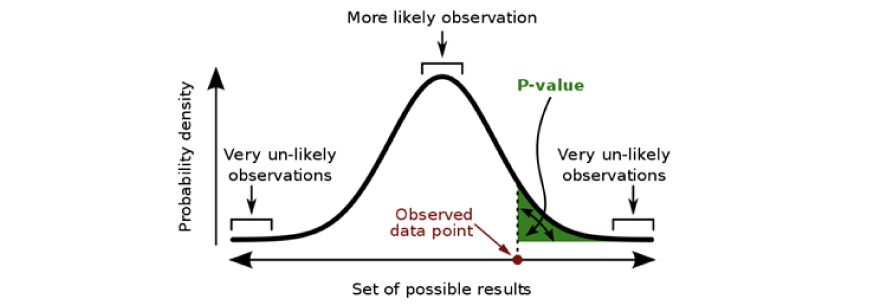Response

A type I error is the incorrect rejection of a true null hypothesis. Typically, a type I error leads one to conclude that a supposed effect or relationship exists when in fact it doesn't.

Examples of type I errors include a test that shows a patient to have a disease when in fact the patient does not have the disease, a fire alarm going on indicating a fire when in fact there is no fire, or an experiment indicating that a medical treatment should cure a disease when in fact it does not.

• Type I error refers to “false positive”.
• A type II error is the failure to reject a false null hypothesis.

Examples of type II errors would be a blood test failing to detect the disease it was designed to detect, in a patient who really has the disease; a fire breaking out and the fire alarm does not ring; or a clinical trial of a medical treatment failing to show that the treatment works when really it does.

Type II error refers to “false negative”.

Response:

This depends on the situation of the context and the data and domain that we are considering and trying to solve.

For email spam filtering use case, a false positive occurs when spam filtering or blocking techniques incorrectly classify a legitimate email message as spam. While most anti-spam techniques can block a high percentage of unwanted emails, doing so without creating significant false-positive outcomes is a much more demanding activity. Hence, we prefer too many false negatives over many false positives.

In another example of a medical testing scenario, false negatives may provide a falsely reassuring message to patients and physicians that disease is absent when it is present. This sometimes leads to inappropriate treatment of both the patient and their associated disease. Hence, it is desired to have too many false positives in this context.

Response:

Imbalanced data usually refers to a problem with classification problems where the classes are not represented equally. For example, in a credit card fraud detection scenario, we may have a 2-class (binary) classification problem with 100 instances (rows). A total of 95 instances are labelled with Class-1 which are genuine transactions and the remaining 5 instances are labelled with Class-2 which are fraudulent transactions.

This is an imbalanced dataset and the ratio of Class-1 to Class-2 instances is 95:5. You can have a class imbalance problem on two-class classification problems as well as multi-class classification problems.

We can handle it in various ways.

1. One way to see if we can collect more data, to make the imbalance cases more balance may be 75:25 split etc.
2. Try to resample your dataset, i.e. over-sampling or under-sampling. We can include copies of instances from the under-represented class called over-sampling. This is similar to formally sampling with replacement. Secondly, we can delete instances from the over-represented class, called under-sampling.
3. We can try changing the performance metric while evaluating the model. Accuracy is not the metric to use when working with imbalanced classes like this example here. Other metrics such as Precision, Recall, F1score, Confusion matrix, etc. can be looked into.
4. We can also try experimenting with different algorithms to see how outcomes differ.

Lot of different aspects can be looked at. All of these vary based on the context, dataset and domain also that we are analyzing on.

Response:

Population is the entire collection of the dataset for which the information is desired. When we deal with the dataset, then the entire set of data which may be a collection of objects or individuals are called as population.

There are different categories of “summary measures”. When we describe data numerically, then we use various summary measures such as the following:

1. Mean
2. Median
3. Quartiles
4. Range
5. Interquartile range (IQR)
6. Variance
7. Standard deviation

Out of the above measures, Mean and Median are used for "centre and location of numerical datasets”. Quartiles are used as other measures of location.  Range, IQR, variance and Standard deviation parameters are used for variances in the dataset.

Response:

Let’s consider a normal distribution N

This is an example of a central limit theorem. As n increases, the average of x (x-bar let's call) should get closer to the population mean (mu) Average of x is denoted as x-bar.

If we consider normal distribution and will try to write below mathematical equation as per normal distribution for this problem:

X ~ N(30, 9.8/sqrt(36))
Probability of a group of objects weighing > 1010 pounds [Symbol]
Prob(x-bar > 1010/36) = Prob[(x-bar – 30) > (1010/36 - 30)] , which further implies:
Prob(x-bar > 1010/36) = Prob[( (x-bar – 30) / (9.8/sqrt(36) ) ) > ((1010/36 – 30) / (9.8/sqrt(36)) )] =>
Prob(x-bar > 1010/36) = Prob(Z > -1.190)
= 1 – 0.1170
= 0.883 (as per normal z-score table)
Hence probability is 88.3% or 0.883 

Here, the probability that z-score > -1.19 is equal to the blue colour area below of area under the curve.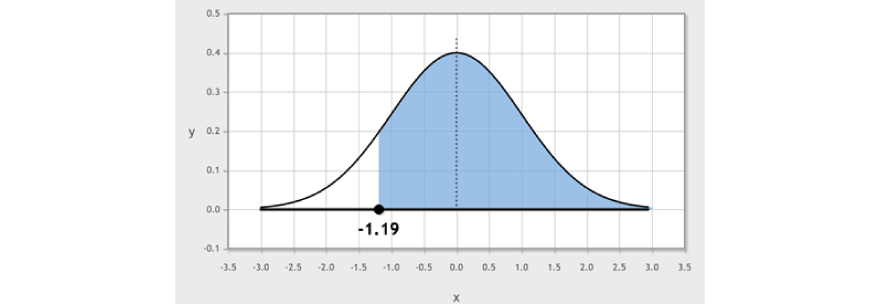Now, the area above -1.19 is same as area below 1.19 as per the distribution diagram below.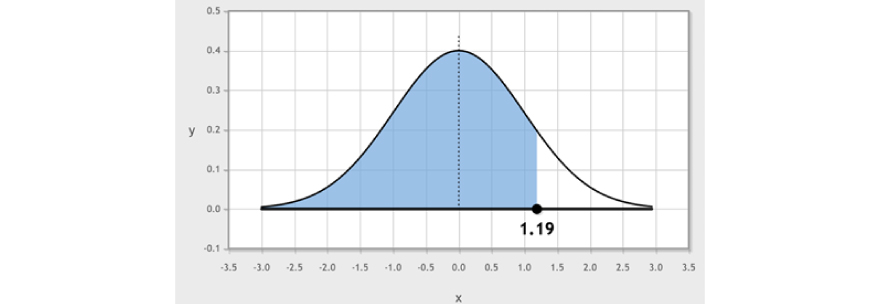Response:

We perform different exploration tasks when we get input datasets to perform analyses and understand the same. Once our data is ready and analyzed, we try to perform the feature engineering process to make it ready to be used in a modelling process.

Feature engineering process comprises of two key tasks such as Variable/feature transformation and Variable/feature creation.

Variable or feature transformation – This is where a variable is replaced by a function. For example, replacing a variable F1 by the square or cube root or logarithm F1 is a transformation. Therefore, this process changes the distribution or relationship of a variable with others.

Some of the situations where we want to use variable transformation are as follows:

1. For standardization purposes or scale-up cases – if it is needed to change the scale of the variable or to standardize it for better interpretation. While transformation is important, it does not necessarily change the shape of a variable distribution.
2. For transforming non-linear to linear relationships – if it is needed to transform non-linear relationships into linear relationships, then this may be used. Linear relationship between variables is simpler to interpret compared to a non-linear relation. Some examples are - Scatter plots, Log transformation approaches. These techniques can be used as different techniques in these situations.

Response:

There are different approaches to transform variables during feature engineering process.

Three methods are explained below:

• Binning approach – variables can be classified or categorized using this approach. This is performed on original values, percentile or frequency of respective variables. Business understanding, goal, objectives are needed to decide on these categorization techniques.
• For example, we can classify income categories in 3 categories, such as High, Average and Low. Anybody with annual income let's say up to 500,000 are into Low category, 500,001 to 20,00,000 falls into Average category and > 20,00,000 falls into High category and so on as an example.
• We can also perform co-variate binning, that depends on the value of more than one variable.
• Log transformation – Log value of a variable is the standard transformation method which is used to change the shape of the distribution of the variable on the particular distribution list. This is generally used for reducing negative skewness of variables. Histograms can be plotted for based kurtosis, mean and standard deviation values, log transformation can be decided.
• Square root or Cube root etc – The square root of a variable is used to have a sound effect on variable distribution. It is not significant compared to Log transformation. Cube root of the variable is used for transformation where it can be applied to negative values including zero.

Response:

Clustering is part of unsupervised learning in machine learning and data science. Cluster analysis or data segmentation is an exploratory method for identifying homogeneous groups or clusters of records.

• Similar records should belong to the same cluster.
• Dissimilar records should belong to different clusters.Clustering algorithms are largely distinguished by two characteristics. One is "similarity metric" and the other is "agglomeration function (kind of merge/bottom up) strategy".

Clustering can be of various types. Some key categories are as follows:

1. Hierarchical clustering – using connectivity models
2. K-means clustering – using centroid models
3. Expectation-maximization – statistics based
4. Density-based – statistics based

Response:

Machine learning can be broadly categorized into the following four types:

1. Supervised
2. Unsupervised
3. Semi-supervised
4. Reinforcement

Below image would provide a very high-level interpretation of different machine learning categories.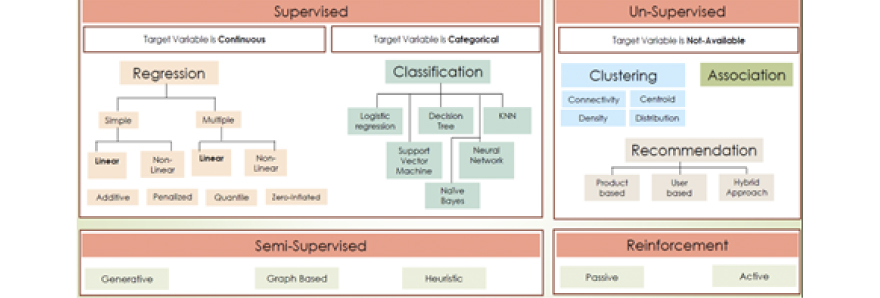Response:

Ensemble methods are based on the idea of combining predictions from many so-called base models. They can be seen as a type of meta-algorithms, in the sense that they are methods composed of other methods.

Bagging, boosting are some key examples of leveraging ensemble methods. Random forest algorithm uses the ensemble approach effectively in specific scenarios.

• Bagging – The idea behind bagging is to train multiple models of the same type in parallel and on different versions of training data. By averaging the predictions of the resulting ensemble of models, it is possible to reduce the variance compared to using only a single model. One of the key implementation examples is Random Forest algorithms in this context. Random forests make use of classification or regression trees as base models. Each tree is randomly perturbed in a certain way which opens up for additional variation reduction in the dataset.
• Boosting – Another approach is boosting which is different than the bagging technique and random forests. Its base models are learned sequentially, one after the other. Hence each model tries to correct for the mistakes done by previous models. By considering the weighted average of the predictions made by base models, this transforms the ensemble of "weak" models into "strong" models.

Response:

Classification and Regression are both used for Supervised Learning cases.

Classification produces discrete values to classify or categorize the target (e.g. fail/No-fail etc.) whereas regression provides a continuous result that allows us to distinguish between various point values effectively.

Hence, in a dataset, if the target variable is continuous, then “regression” will be used. If the target variable is categorical, then “classification” will be used.

If we wanted to predict whether a machine will fail or not in future, we will use classification. If we want to predict the height of a person based on other relative attributes where target is a number and continuous of nature, then we will use regression.

Of course, there are different types of regression and they are not same and have different techniques to solve different type of business problems.

Response:

Cross-validation (CV) is a technique used to validate machine learning models. The data set is divided into training and test datasets. The model is created based on the training dataset and trained on that. It is then used to validate with some new dataset which is a test dataset. Cross-validation is a technique for asserting how results or outcomes of a statistical analysis on a given dataset will generalize to an independent dataset.

A sample representation can be illustrated below.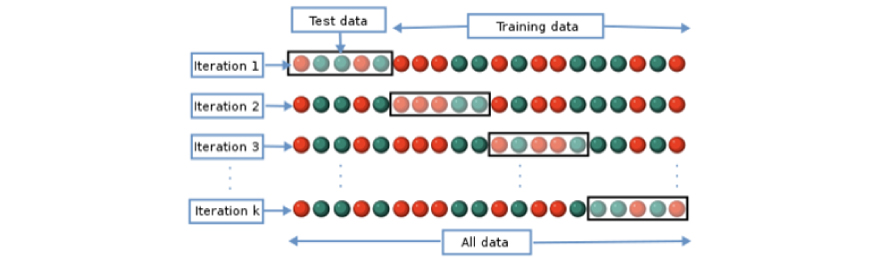Here training and test data are shuffled randomly to create multiple flavours for various iterations. The objective of a CV is to test a model's ability to predict new data that was not used while training the model or estimating the model, to help identify issues such as overfitting or bias etc. Hence the model can be generalized by using certain approaches once we perform CV tests.

5-fold CV is nothing but CVs covering 5 iterations.

This could be represented or illustrated by the below image.Response:

When branches in a decision tree have weak predictive power, they are removed to reduce the complexity of the model or solution. They also increase the predictive accuracy of a decision tree. This is referred to as pruning on decision trees which are basic tree-based approaches used in machine learning.

Reduced error pruning is one of the simple methods that replace each node. This is used for optimizing the accuracy of the model/solution.

An unpruned decision tree example: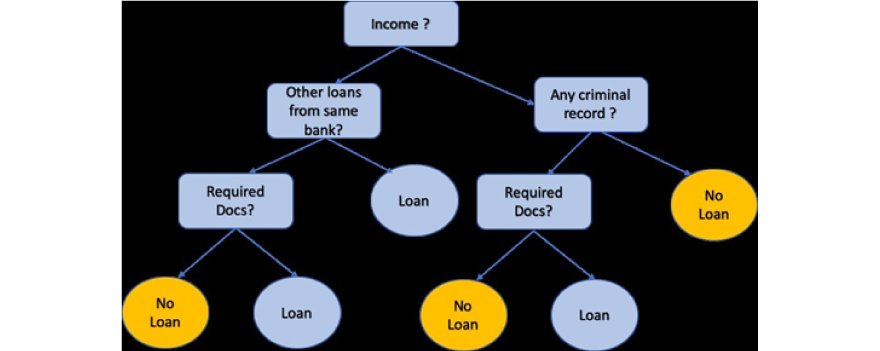A pruned decision tree example: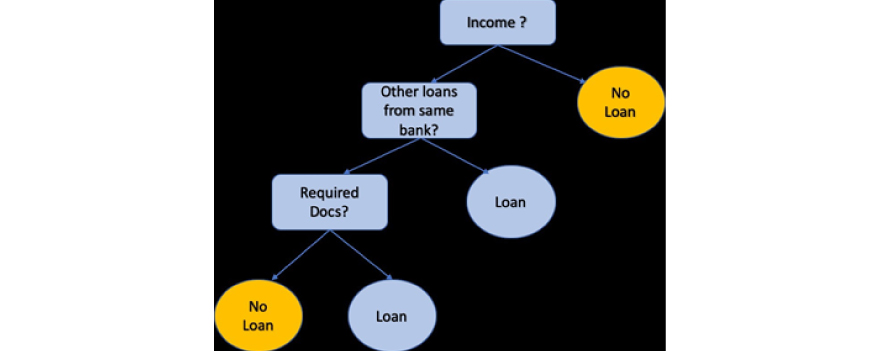A pruned tree has fewer nodes and less sparsity compared to an unpruned tree.

Response:

Machine Learning interpretability refers to a concept where one can have a better understanding of what is happening as part of the predicted outcome from an ML model. In real-world scenarios, there are always data quality issues, nature of data distribution, the way data has been collected or gathered over some time etc. has a lot of impact in formulating the machine learning process in which the model is developed. The outcome of a model in terms of prediction accuracy or something similar largely depends on various aspects such as features that are used, variation in features, data distribution, variation of correlation between those features etc.

There are different types of dataset shifts. These are critical since this will impact the model performance after it is being put into production. Existing model, which is trained and developed in the development phase, may change due to various factors.

1. Covariate shift – when a shift occurs in independent variables, then it is termed as covariate shift.
2. Concept shift – when a shift occurs between the relationship of independent and target variables, then it is termed as concept drift.
3. Prior probability shift - when a shift occurs in target variable, then it is termed as prior probability shift.

Response:

As we understand, dataset shift is a problem when we put our model from development environment to production environment. These of course are classified into various types depending on whether there is a shift that occurs later between an independent and target variable, or within independent variables or with the target variable only.

The causes for dataset shift can be due to the following factors:

1. Production model is no longer fit for purpose because of changes due to data distribution, variation in data parameters/features etc.
2. May be difficult to detect if there is any such dataset shift
3. There is an inherent need to monitor models that are in production regularly to ensure model performance does not degrade
4. Changes in behaviour of model features may be sequential, gradual or ad-hoc (depending on data quality and how data changes over some time)
5. Increased model maintenance

Following attempts can be taken to address these issues at hand.

1. Re-fit or upgrade the model periodically based on a certain frequency by checking model performance or accuracy (e.g. checking Precision / Recall for a certain scenario against new data for few weeks)
2. Keep monitoring distribution of independent variables in the dataset in the production environment.
3. Keep assessing the model performance periodically.
4. Weight the data
5. Learn the change in features in the dataset.

Response:

Principal Component Analysis (PCA) is a dimensionality reduction technique used in Machine learning. That means, it is an approach to extract or detect key features (in the form of components) from the input dataset which may have a large set of features. Hence it is a kind of a feature selection method.

The objective is to select a few features or variables that represent as much information as can be possible for us to be able to use those for the learning process.

Hence it is used to overcome redundancy in features in the dataset and by identifying those, a decision can be taken to optimize them or drop them. This method is generally applied on datasets with numbers.

Response:

Model evaluation techniques are as follows:

1. AUC (Area under the curve) – ROC (Receiver Operating Characteristic)
2. Precision and Recall charts and F1 score
3. KS Chart or Kolmogorov Smirnov Chart
4. RMSE or Root Mean Square Error
5. MAPE or (Mean Absolute Percentage Error)
6. Gini Coefficient

Out of the above, RMSE and MAPE can be used to evaluate linear regression models or algorithms.

Response:

Gini Coefficient is a measure to use feature importance in a random forest algorithm. In a binary classification scenario, when we are predicting both classes, the Gini coefficient can be computed based on AUC (Area Under the Curve) value.

It measures the inequality between values of a frequency distribution.

It is computed as the following:

Gini Coeff (Gini Coefficient) = 2 * AUC – 1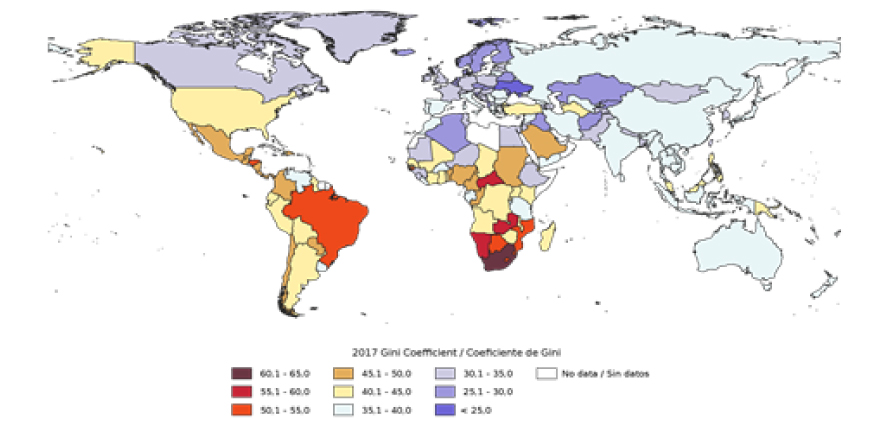In the above example and figure 25, Gini Coeff = A / (A+B).

Wikipedia defines word embedding as collective name for a set of language modeling and feature learning techniques in natural language processing (NLP) where words or phrases from the vocabulary are mapped to vectors of real numbers. Word embeddings are a way to transform words in text to numerical vectors so that they can be analysed by standard machine learning algorithms that require vectors as numerical input.

Now vectorisation can be done in many ways – One-hot-encoding, Latent Semantic Analysis (LSA),TF-IDF (Term Frequency, Inverse document frequency etc.). However, these representations capture a slightly different document-centric idea of semantic similarity.

Distributed Representation :

Distributed representations attempt to capture the meaning of a word by considering its relations with other words in its context. The idea is captured in this quote from J. R. Firth (for more information refer to the article: Document Embedding with Paragraph Vectors, by Andrew M. Dai, Christopher Olah, and Quoc V. Le, arXiv:1507.07998, 2015), a linguist who first proposed this idea: “You shall know the word by the company it keeps”.

Consider the following pair of sentences:

Paris is the capital of France. Berlin is the capital of Germany.

Even assuming you have no knowledge of world geography (or English for that matter), you would still conclude without too much effort that the word pairs (Paris, Berlin) and (France, Germany) were related in some way, and that corresponding words in each pair were related in the same way to each other, that is:

Paris : France :: Berlin : Germany

Thus, the aim of distributed representations is to find a general transformation function φ to convert each word to its associated vector such that relations of the following form hold true:

Word2vec:

The word2vec group of models was created in 2013 by a team of researchers at Google led by Tomas Mikolov. The models are basically unsupervised, taking as input a large corpus of text and producing a vector space of words. The dimensionality of the word2vec embedding space is usually lower than the dimensionality of the one-hot embedding space, which is the size of the vocabulary. The embedding space is also more dense compared to the sparse embedding of the one-hot embedding space.

The two architectures for word2vec are as follows:

• Continuous Bag Of Words (CBOW)
• Skip-gram

In the CBOW architecture, the model predicts the current word given a window of surrounding words. In addition, the order of the context words does not influence the prediction (that is, the bag of words assumption). In the case of skip-gram architecture, the model predicts the surrounding words given the centre word. According to the authors, CBOW is faster but skip-gram does a better job at predicting infrequent words.

For various distance-based measures like KNN (K-Nearest neighbour) method, the performance or predictive power of the model deteriorates with the increase in numbers of features required for prediction. This is an obvious fact that high- dimensional spaces are vast. Points in high-dimensional spaces tend to be dispersing from each other more compared with the points in low-dimensional space.

It is apparent that with the increase in dimensions, distance between points increases logarithmically, which gives us the hint that we need to have an exponential increase in data points with the increase in dimensions in order to make machine learning algorithms work correctly.

It can be proved that with the increase in dimensions, mean distance increases logarithmically. Hence the higher the dimensions, the more data is needed to overcome the curse of dimensionality!

Box-Cox transform function belongs to the Power Transform family of functions. These functions are primarily used to create monotonic data transformations, but their main significance lies in the fact that they help in stabilizing variance by adhering closely to the normal distribution and making the data independent of the mean based on its distribution. This function has one prerequisite that the numeric values to be transformed must be positive (similar to what even log transform expects). In case they are negative, shifting using a constant value helps. Mathematically, the Box-Cox transform function can be defined as:Such that the resulted transformed output y is a function of input x and transformation parameter λ such that when λ= 0, the resultant transform is the natural log transform, which we discussed earlier. The optimal value of λ is usually determined using a maximum likelihood or log-likelihood estimation.

Data Come in various shapes and sizes, and measure different things at different times. Financial analysts are often interested in particular types of data, such as time-series data or cross-sectional data or panel data.

• Time Series Data: A time series dataset is one where the observations are time-dependent. For instance, let us now suppose that a researcher collects salary data across a city on a month-by-month basis. The observations in the dataset will now differ at various  time points.
• Cross – Sectional Data: A cross-sectional dataset is one where all data is treated as being at one point in time. Let's consider that you have a dataset of salaries across a city - they have all been gathered at one point in time and thus we refer to the data as cross-sectional.
• Panel Data: Pooled (or panel) data is where the two are combined together. i.e. a salary dataset can contain observations collected at one point in time, as well as across different time periods.

Few additional points to bear in mind in this regard – The most common issues when working with cross-sectional data are multicollinearity and heteroscedasticity. Multicollinearity is where two or more independent variables are correlated with each other. Heteroscedasticity is where the variance of the error term is not constant (e.g. salaries are typically higher in bigger vs. smaller cities, skewing results towards bigger cities).

For time series data, serial correlation (also known as autocorrelation) is an issue. This happens when correlations exist across the error term across different time periods. e.g. if salaries are growing across time as a worker gets more experience, this does not allow us to identify important differences between salaries across different observations.

Various methods and techniques are there to deal with each of these problems.

A type of stochastic process that has received a great deal of attention and scrutiny by time series analysts is the so-called stationary stochastic process. Broadly speaking, a stochastic process is said to be stationary if its mean and variance are constant over time and the value of the covariance between the two time periods depends only on the distance or gap or lag between the two time periods and not the actual time at which the covariance is computed. In the time series literature, such a stochastic process is known as a weakly stationary, or covariance stationary, or second-order stationary, or wide sense, stochastic process.

In short, if a time series is stationary, its mean, variance, and autocovariance (at various lags) remain the same no matter at what point we measure them; that is, they are time invariant. Such a time series will tend to return to its mean (called mean reversion) and fluctuations around this mean (measured by its variance) will have a broadly constant amplitude.7 If a time series is not stationary in the sense just defined, it is called a nonstationary time series (keep in mind we are talking only about weak stationarity). In other words, a nonstationary time series will have a time-varying mean or a time-varying variance or both.

Why are stationary time series so important? Because if a time series is nonstationary, we can study its behaviour only for the time period under consideration. Each set of time series data will therefore be for a particular episode. As a consequence, it is not possible to generalize it to other time periods. Therefore, for the purpose of forecasting, such (nonstationary) time series may be of little practical value.

There are various ways to study non-stationarity of time series data – Augmented Dicky Fuller (ADF) test one of those very popular test to determine the nature of stationarity.

The main goals of power analysis are two folds in the process of designing an experiment, (a) how large a sample is required for making statistical judgments that are accurate and reliable and (b) how likely your statistical test will be to detect effects for a given size in a particular situation.

In other words, Power analysis is a very crucial aspect of experimental design. It helps us to determine the sample size required to detect an effect of a given size with a given degree of confidence. Conversely, it allows us to determine the probability of detecting an effect of a given size with a given level of confidence, under sample size constraints. If the probability is unacceptably low, we would be advised to alter or abandon the experiment.

The following four quantities are most important so far Power analysis is concerned:

1. sample size
2. effect size
3. significance level = P(Type I error) = probability of finding an effect that is not there
4. power = 1 - P(Type II error) = probability of finding an effect that is there

Given any three, we can determine the fourth.

The LR model is based on certain assumptions, some of which refers to the distribution of the random variable (error term : e) and finally some refer to the relationship between e and the explanatory variables. We will group them in two categories (i) Stochastic Assumptions (ii) Other assumptions.

• Stochastic Assumptions:
• ei is a random real variable.
• The mean value of “e” in any particular period is zero.
• The variance of ei is constant in each period ( This is sometimes referred as assumption on “Homoscedastic” Variance).
• The variable ei has a normal distribution.
• The random terms of different observations (ei, ej) are statistically independent (no auto-correlation among error terms).
• “e” is independent of the explanatory variable(s) (X).
• The explanatory variables are measured without error.
• The Xi’s are set of fixed values in the hypothetical process of repeated sampling which underlies the LR model.
• Other Assumptions:
• The explanatory variables are not perfectly linearly correlated.
• The macro variables should be correctly aggregated.
• The relationship being estimated is identified.
• The relationship is correctly specified.

(Please refer to the Book – “The theory of econometrics – 2nd Edition by A. Koutsoyiannis”)

As Francois Chollet in his book “Deep Learning with Python” has defined “Deep learning is a specific subfield of machine learning: a new take on learning representations from data that puts an emphasis on learning successive layers of increasingly meaningful representations.” The deep in deep learning does not necessarily refer to any kind of “deeper understanding achieved by the approach; rather, it stands for the idea of successive layers of representations. How many layers contribute to a model of the data is called the depth of the model.

Other appropriate names for the field could have been layered representations learning and hierarchical representations learning. Modern deep learning often involves tens or even hundreds of successive layers of representations— and they’ve all learned automatically from exposure to training data. Meanwhile, other approaches to machine learning tend to focus on learning only one or two layers of representations of the data; hence, they’re sometimes called shallow learning.” (Please refer to the Book – “Deep Learning with Python” by Francois Chollet)

Reinforcement Learning is a special brunch of Machine Learning that has received a lot of attention in recent times after Google DeepMind successfully applied it to learning to play Atari games (and, later, learning to play Go at the highest level). Typically RL refers to a framework where an agent receives information about its environment and learns to choose actions that will maximize some reward. For instance, a neural network that “looks” at a videogame screen and outputs game actions in order to maximize its score can be trained via reinforcement learning.

Currently, reinforcement learning is one of the most researched area and yet to be significantly successful beyond games. In time, however, we expect to see reinforcement learning take over an increasingly large range of real-world applications: self-driving cars, robotics, resource management, education, and so on. It’s an idea whose time has come, or will come soon.

One approach would be to calculate “Point Bi-serial Correlation” which will give you an estimate to measure the degree of association between a binary variable and continuous variable. The Point-Biserial Correlation Coefficient is a correlation measure of the strength of association/coherence between a continuous-level variable (ratio or interval data) and a binary variable.  Binary variables are variables of nominal scale with only two values.  They are also called dichotomous variables or dummy variables in Regression Analysis.

Mathematically, the Point-Biserial Correlation Coefficient is calculated just as the Pearson’s Bivariate Correlation Coefficient would be calculated, wherein the dichotomous variable of the two variables is either 0 or 1—which is why it is also called the binary variable.  Since we use the same mathematical concept, we do need to fulfil the same assumptions, which are normal distribution of the continuous variable and homoscedasticity.

An ROC curve (receiver operating characteristic curve) is a graph showing the performance of a classification model at all classification thresholds. This curve basically plots two parameters:

• True Positive Rate/Recall – TPR = TP/TP+FN
• False Positive Rate – FPR = FP/FP+TN

An ROC curve plots TPR and FPR at different classification/probability thresholds. Lowering the classification threshold classifies more items as positive, thus increasing both False Positives and alternatively True Positives. In order to compute the points in an ROC curve, one could evaluate a logistic regression model many times with different classification thresholds, but this would be inefficient. Fortunately, there's an efficient, sorting-based algorithm that can provide this information for us, called AUC.

AUC stands for "Area under the ROC Curve." i.e. , AUC measures the two-dimensional area underneath the entire ROC curve ranging from (0,0) to (1,1). AUC provides an aggregate measure of performance across all possible classification thresholds. One way of interpreting AUC is as the probability that the model ranks a random positive example more highly than a random negative example.

AUC ranges in value from 0 to 1. A model whose predictions are 100% incorrect has an AUC of 0.0; one whose predictions are 100% correct has an AUC of 1.0.

If we have two different probability distributions P(x) and Q(x) over the same random variable x, we can measure how diﬀerent these two distributions are using the Kullback-Leibler (KL) divergence:In the case of discrete variables, it is the extra amount of information (measured in bits if we use the base-2 logarithm, but in machine learning we usually use nats and the natural logarithm) needed to send a message containing symbols drawn from probability distribution P, when we use a code that was designed to minimize the length of messages drawn from probability distribution Q. The KL divergence has many useful properties, most notably being non-negative. The KL divergence is 0 if and only if P and Q are the same distribution in the case of discrete variables, or equal “almost everywhere” in the case of continuous variables. Because the KL divergence is non-negative and measures the diﬀerence between two distributions, it is often conceptualized as measuring some sort of distance between these distributions.

One use for KL-divergence in the context of discovering correlations is to calculate the Mutual Information (MI) of two variables which can reveal some pattern between two different variables and provide idea about the correlation structure.

Another use for Kullback-Leibler divergence is in the domain of variational inference, where an optimization problem is constructed that to minimize the KL-divergence between the intractable target distribution P and a sought element Q from a class of tractable distributions.

Many approximating algorithms (which can also be used to fit probabilistic models to data) can be interpreted using KL divergence. Among those are Mean Field, (Loopy) Belief Propagation (generalizing forward-backward and Viterbi for HMMs), Expectation Propagation, Junction graph/tree, tree-reweighted Belief Propagation.

(Please refer to: Wainwright, M. J. and Jordan, M. I. Graphical models, exponential families, and variational inference, Foundations and Trends text registered in Machine Learning, Now Publishers Inc., 2008, Vol. 1(1-2), pp. 1-305)

One of the key steps in building a machine learning model is to estimate its performance on data that the model hasn't seen before. Let's assume that we t our model on a training dataset and use the same data to estimate how well it performs on new data.

A typical model may either suffer from underfitting (high bias) if the model is too simple, or it can overfit if the training data (high variance) if the model is too complex for the underlying training data. To find an acceptable bias-variance trade-off, we need to evaluate our model carefully. In this section, you will learn about the common cross-validation techniques holdout cross-validation and k-fold cross-validation, which can help us obtain reliable estimates of the model's generalization performance, that is, how well the model performs on unseen data.

The Holdout Method:

A classic and popular approach for estimating the generalization performance of machine learning models is holdout cross-validation. Using the holdout method, we split our initial dataset into a separate training and test dataset—the former is used for model training, and the latter is used to estimate its generalization performance. However, in typical machine learning applications, we are also interested in tuning and comparing different parameter settings to further improve the performance for making predictions on unseen data.

A disadvantage of the holdout method is that the performance estimate may be very sensitive to how we partition the training set into the training and validation subsets; the estimate will vary for different samples of the data.

The K-fold cross validation Method:

In k-fold cross-validation, we randomly split the training dataset into folds without replacement, where — 1 folds are used for the model training, and one fold is used for performance evaluation. This procedure is repeated times so that we obtain models and performance estimates. We then calculate the average performance of the models based on the different, independent folds to obtain a performance estimate that is less sensitive to the sub-partitioning of the training data compared to the holdout method. Typically, we use k-fold cross-validation for model tuning, that is, finding the optimal hyperparameter values that yields a satisfying generalization performance.

Since k-fold cross-validation is a resampling technique without replacement, the advantage of this approach is that each sample point will be used for training and validation (as part of a test fold) exactly once, which yields a lower-variance estimate of the model performance than the holdout method.

A good standard value for in k-fold cross-validation is 10, as empirical evidence shows. For instance, experiments by Ron Kohavi on various real-world datasets suggest that 10-fold cross-validation offers the best trade-off between bias and variance (A Study of Cross-Validation and Bootstrap for Accuracy Estimation and Model Selection, Kohavi, Ron, International Joint Conference on Arti cial Intelligence (IJCAI), 14 (12): 1137-43, 1995).

A special case of k-fold cross-validation is the Leave-one-out cross-validation (LOOCV) method. In LOOCV, we set the number of folds equal to the number of training samples (k = n) so that only one training sample is used for testing during each iteration, which is a recommended approach for working with very small datasets.

Following methods can be considered for finding optimal value of K in K-means clustering.

Approximate Expected Overall R-square:  Approximate Expected Overall R-Square is calculated based on the hypothesis that all the explanatory variables used for Clustering are independent. Hence if there is a lot of difference between Observed Overall R-square and Approximate Expected Overall R-square, we can suspect high correlation among the independent variables.

Cubic Clustering Criterion:

• Comparative measure of the deviation of the clusters from the distribution expected if data points were obtained from a uniform distribution
• Larger positive values of the CCC indicate a better solution, as it shows a larger difference from a uniform (no clusters) distribution.
• Large negative Values indicate the presence of Outliers

Pseudo F:

• The pseudo F statistic measures the separation among all the clusters at the current level
• Relatively large values indicate a stopping point. Reading down the PSF column, find all possible stopping points (where PSF is very large compared to other values).

The optimal number of clusters is found at a point where CCC and Pseudo-F reach maximum and Overall R-Square tapers off.

Elbow Method: The Elbow method is a method of interpretation and validation of consistency within cluster analysis designed to help finding the appropriate number of clusters in the data set. One simple heuristic is to compute the total within sum of squares (WSS) for different values of k and look for an “elbow” in the curve. Define the cluster’s centroid as the point that is the mean value of all the points in the cluster. The within sum of squares for a single cluster is the average squared distance of each point in the cluster from the cluster’s centroid. The total within sum of squares is the sum of the within sum of squares of all the clusters. The total WSS will decrease as the number of clusters increases, because each cluster will be smaller and tighter. The hope is that the rate at which the WSS decreases will slow down for k beyond the optimal number of clusters. In other words, the graph of WSS versus k should flatten out beyond the optimal k, so the optimal k will be at the “elbow” of the graph. Unfortunately, this elbow can be difficult to see.

CH Index (Calinski-Harabasz): The Calinski-Harabasz index of a clustering is the ratio of the between-cluster variance (which is essentially the variance of all the cluster centroids from the dataset’s grand centroid) to the total within-cluster variance (basically, the average WSS of the clusters). For a given dataset, the total sum of squares (TSS) is the squared distance of all the data points from the dataset’s centroid. The TSS is independent of the clustering. If WSS(k) is the total WSS of a clustering with k clusters, then the between sum of squares BSS(k) of the clustering is given by BSS(k) = TSS - WSS(k). WSS(k) measures how close the points in a cluster are to each other. BSS(k) measures how far apart the clusters are from each other. A good clustering has a small WSS(k) and a large BSS(k).The within-cluster variance W is given by WSS(k)/(n-k), where n is the number of points in the dataset. The between-cluster variance B is given by BSS(k)/(k-1). The within-cluster variance will decrease as “K” increases; the rate of decrease should slow down past the optimal k. The between-cluster variance will increase as k, but the rate of increase should slow down past the optimal k. So in theory, the ratio of B to W should be maximized at the optimal k.

All these metrics can be evaluated to decide on the final value for K.

## Description

Being a Data Scientist is not an easy role to get into. Also just having a degree in mathematics/engineering is not enough, a data scientist also needs to develop all the skills mandated by the industry. If you are aspiring to become a Data Scientist but finding it difficult to crack the interview, these Data Science interview questions will be helpful for you.

These top Data Science Interview Questions and Answers will prepare for Data Science interview. If you are already working in Data Science projects and you want to learn Python and R programming language to increase your skill-set, you can still practice these interview questions and answers for Data Science. Preparing these Data Science interview questions will increase your visibility to the potential employers.